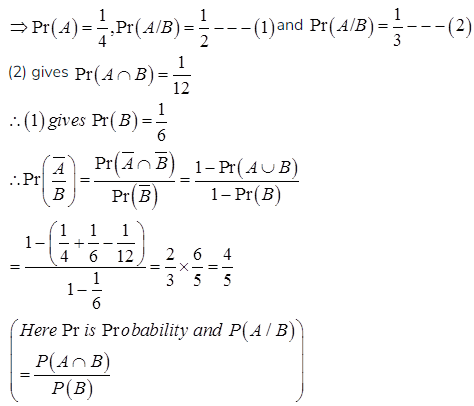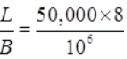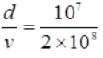# GATE Solved Paper 2017-19 - GATE 2017 Shift 2

>>>>>>>>GATE 2017 Shift 2

• Option : A
• Explanation :
Let A, B be the events denote that P, Q respectively applies for a job• A

2• B

3• C

4• D

5• Option : D
• Explanation :
Since one eigen value of M is 2 thus it satisfies the characteristics equation so
∴ 23 - 4(2)2 + a(2) + 30 = 0
⇒ a = -11
∴ Charcteristic polynomial is
λ3 - 4λ2 - 11λ + 30 = 0
(λ - 2)(λ - 5)(λ + 3) = 0
∴ λ=2,5,-3
Largest absolute value of 'λ' is 5

• A

E -> E - T | T
T -> T + F | F
F -> (E) | id• B

E -> TE'
E' -> -TE' | ε
T -> T + F | F
F -> (E) | id• C

E -> TX
X -> -TX | ε
T -> FY
Y -> +FY | ε
F -> (E) | id• D

E -> TX | (TX)
X -> -TX | +TX | ε
T -> id• Option : C
• Explanation :
The rule for removal of left recursion is
A →Aα |β will be
A →β A’
A’ → αA’|ϵ
The given grammar is:
E → E – T | T; in this α is “– T” and β is T
T →T + F | F, In this α is “+ F” and β is F
F → (E) | id
Hence after removal of the left recursion:
E → TX
X → - TX|ϵ
T → FY
Y → +FY|ϵ
F → (E) | id

• A

0.111 and 0.056• B

0.056 and 0.111• C

0.0892 and 0.1784• D

0.1784 and 0.0892• Option : A
• Explanation :
--Access time of L1 = 1
--Access Time of L2=8
--miss penalty L1 cache (2*L2) = 18*2 = 2*a
--miss penalty L2 cache say a = 18
--AMAT (average memory access time) =2
AMAT = Access time of L1 + (MissRate L1 * miss penalty L1) where miss penalty L1 = Access time of L2 + (MissRate L2 * miss penalty L2)
2 = 1+ 2*a *(8 + a* 18)
Solving the equation,
a=0.111

• A

p = 50 and q = 100• B

p = 50 and q = 400• C

p = 100 and q = 50• D

p = 400 and q = 50• Option : D
• Explanation :B = 106bits/sec
d = 10,000km = 104 x 10 3m
V = 2 x 108 m/s
L = 50,000 Bytes
∴ Transmission time (p) == 400 ms
∴ Propagation time (q) == 50 ms
Related Quiz.
GATE 2017 Shift 2